1.The diagrams show four arrangements of resistors.Which arrangement has the smallest total resistance?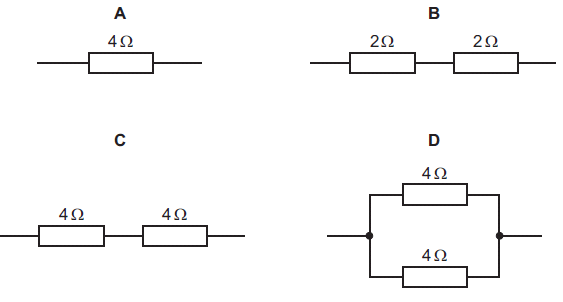2.The potential divider shown is connected across a constant 12 V supply.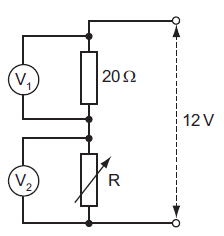When R has a value of 20 ƒ¶, the voltmeter readings are equal. How do these readings change when the value of R is reduced to 10 ƒ¶?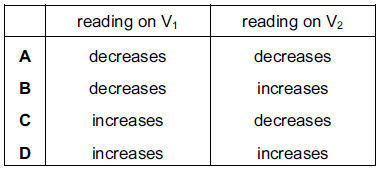3.A circuit contains two resistors connected in parallel with a battery.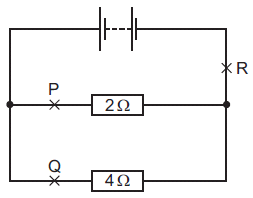Which of the following statements about the currents at P, Q and R is true?

4.The diagram shows a circuit containing two ammeters and three resistors.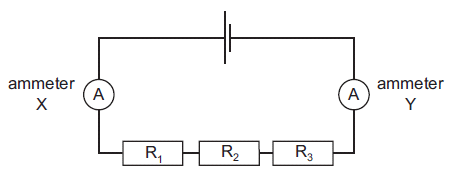Which of the ammeters will show the current in resistor R2?

5.Which copper wire would have the smallest resistance?

6.The reading on the ammeter in the circuit is 1.0 A. A second ammeter is connected in the circuit. It also reads 1.0 A. At which labelled point is it connected?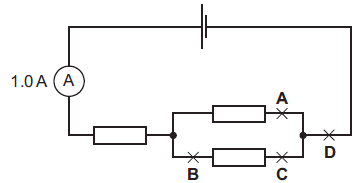7.The diagram shows an electric circuit containing three meters, X, Y and Z, all connected correctly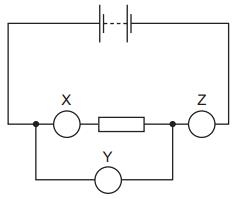What are meters X, Y and Z?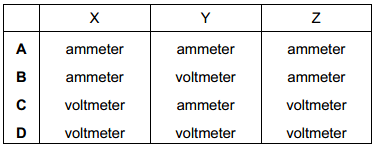8.. The diagram shows a potential divider circuit with two identical lamps L1and L2.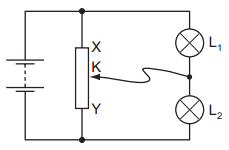The contact K is halfway between X and Y and the lamps are equally bright. What will happen to the brightness of the lamps when contact K is moved a short distance towards X?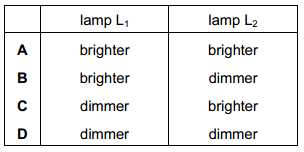9.The diagrams show four arrangements of resistors. Which arrangement has the LARGEST total resistance?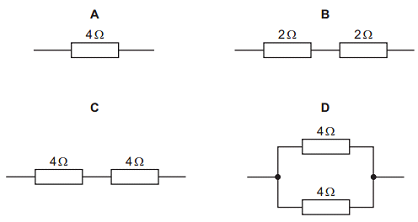10. A resistor in a circuit has a value of resistance of 3.0Ω. In 20 s, a charge of 10 C passes through the resistor. What is the potential difference across the resistor?

Question 1 of 10